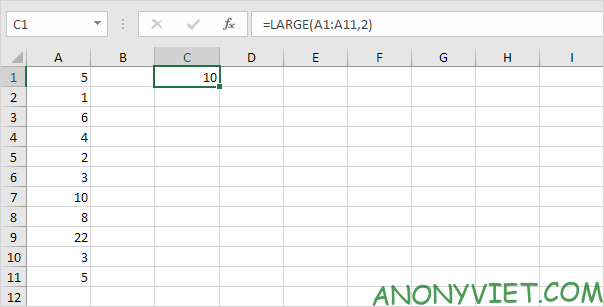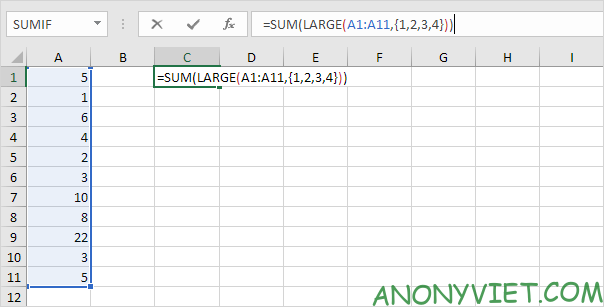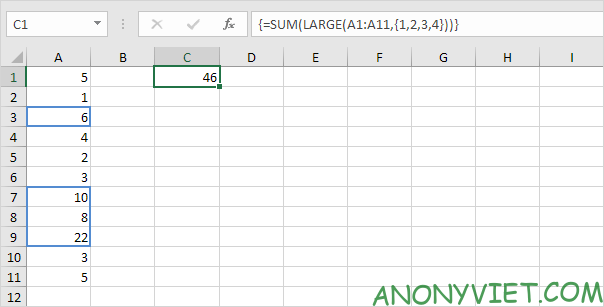# Lesson 205: Sum the largest numbers in Excel

This article shows you how to create an array formula that sums the largest numbers in an Excel range.

 Join the channel Telegram belong to AnonyViet 👉 Link 👈

## Sum the largest numbers in Excel

1. We use the LARGE function. For example, to find the second largest number, use the following function.2. To sum the 4 largest numbers, add the SUM function and replace 2 with {1,2,3,4}.3. Finish by pressing CTRL + SHIFT + ENTER.Note: The formula bar indicates this is an array formula by enclosing it in curly braces {}. Don’t type these yourself. They will disappear when you edit the formula.

Explanation: The range (array constant) created by the LARGE function is stored in Excel’s memory, not in a range. The array constant looks like this.

{22,10,8,6}

This array constant is used as a parameter to the SUM function, giving the result 46.

In addition, you can also see many other excel articles here.

The article achieved: 5/5 – (100 votes)

Source: Lesson 205: Sum the largest numbers in Excel
– TechtipsnReview